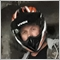# HELP ...Change multiplier lot to +155

Hi, i need to chage the multiplier by increasing +1.

like this 0.01,0.02,0.03,0.04...++

double NR(double thelot)

{

double maxlots = MarketInfo(Symbol(), MODE_MAXLOT),

minilot = MarketInfo(Symbol(), MODE_MINLOT),

lstep = MarketInfo(Symbol(), MODE_LOTSTEP);

double lots = lstep * NormalizeDouble(thelot / lstep, 0);

lots = MathMax(MathMin(maxlots, lots), minilot);

return (lots);

}

double xlot(int m)

{

for (int i = 0; i < OrdersTotal(); i++)

{

continue;

if (OrderSymbol() != Symbol() || OrderMagicNumber() != Magic ||OrderType()!=m)

continue;

dlot=OrderLots();

}

if(OrderType()==m)

//ylot=NR(Lots*MathPow(Multiplier,jumlahorder(m)));                     <<<------------this part how do i change that?

return(ylot);

}38714

Congrats.55

Alain Verleyen:
Congrats.
thank you ;), are you able to help?15338

Hi, i need to chage the multiplier by increasing +1.

like this 0.01,0.02,0.03,0.04...++

double NR(double thelot)

{

double maxlots = MarketInfo(Symbol(), MODE_MAXLOT),

minilot = MarketInfo(Symbol(), MODE_MINLOT),

lstep = MarketInfo(Symbol(), MODE_LOTSTEP);

double lots = lstep * NormalizeDouble(thelot / lstep, 0);

lots = MathMax(MathMin(maxlots, lots), minilot);

return (lots);

}

double xlot(int m)

{

for (int i = 0; i < OrdersTotal(); i++)

{

continue;

if (OrderSymbol() != Symbol() || OrderMagicNumber() != Magic ||OrderType()!=m)

continue;

dlot=OrderLots();

}

if(OrderType()==m)

//ylot=NR(Lots*MathPow(Multiplier,jumlahorder(m)));                     <<<------------this part how do i change that?

return(ylot);

}

Hi,

double Last_lot =Last_lot(); //get

double min=SymbolInfoDouble(Symbol(),SYMBOL_VOLUME_MIN);
double Lotstep=SymbolInfoDouble(Symbol(),SYMBOL_VOLUME_STEP);
int    LotsDigits=(int)MathCeil(MathAbs(MathLog(Lotstep)/MathLog(10)));

// if(initial_lot<Lotstep) initial_lot=Lotstep;

double next_lot=NormalizeDouble(Last_lot+initial_lot,LotsDigits);20233

amerul.trader: Hi, i need to chage the multiplier by increasing +1. like this 0.01,0.02,0.03,0.04...++
`      //ylot=NR(Lots*MathPow(Multiplier,jumlahorder(m)));                     <<<------------this part how do i change that?`
1. That not a multiplier, it's an increment.

2. Please edit your (original) post and use the CODE button (Alt-S)! (For large amounts of code, attach it.)
General rules and best pratices of the Forum. - General - MQL5 programming forum
Messages Editor

3. You haven't stated a problem, you stated a want. Show us your attempt (using the CODE button) and state the nature of your problem.
No free help
urgent help.

Or pay someone. Top of every page is the link Freelance.